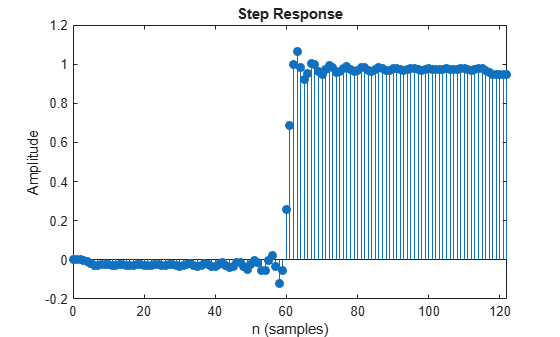# stepz

Step response of discrete-time filter System object

## Syntax

``````[stepResp,t] = stepz(sysobj)``````
``````[stepResp,t] = stepz(sysobj,n)``````
``````[stepResp,t] = stepz(sysobj,n,fs)``````
``````[stepResp,t] = stepz(sysobj,[],fs)``````
``````[___] = stepz(___,'Arithmetic',arithType)``````
``stepz(sysobj)``

## Description

``````[stepResp,t] = stepz(sysobj)``` computes the step response of the filter System object™ and returns the response in the column vector `stepResp`, and a vector of times (or sample intervals) in `t`, where ```t = [0 1 2 ...k-1]'```. `k` is the number of filter coefficients.```
``````[stepResp,t] = stepz(sysobj,n)``` computes the step response at `floor(n)` 1-second intervals. The time vector `t` equals `(0:floor(n)-1)'`.```
``````[stepResp,t] = stepz(sysobj,n,fs)``` computes the step response at `floor(n)` 1/`fs`-second intervals. The time vector `t` equals `(0:floor(n)-1)'/fs`.```
``````[stepResp,t] = stepz(sysobj,[],fs)``` computes the step response at `k` 1/`fs`-second intervals, where `k` is the number of filter coefficients. The time vector `t` equals `(0:k-1)'/fs`.```
``````[___] = stepz(___,'Arithmetic',arithType)``` analyzes the filter System object based on the arithmetic specified in `arithType` using any one of the previous syntaxes.```

example

````stepz(sysobj)` launches `fvtool` and plots the step response of the filter System object.For more input options, see `stepz` in Signal Processing Toolbox™.`stepz` works for both real and complex filters. When you omit the output arguments, the `stepz` function plots only the real part of the step response.```

## Examples

collapse all

Design an equiripple lowpass FIR filter and compute the step response of the filter.

Use the `fdesign.lowpass` specification object to define the filter specifications. Run the `design` function on the specification object to create a `dsp.FIRFilter` object. Specify the passband frequency to be 20 kHz, stopband frequency to be 22.05 kHz, passband ripple to be 1 dB, and the stopband attenuation to be 80 dB. Specify the sampling frequency to be 96 kHz.

```Fs = 96e3; filtSpecs = fdesign.lowpass(20e3,22.05e3,1,80,Fs); firlp2 = design(filtSpecs,'equiripple','SystemObject',true)```
```firlp2 = dsp.FIRFilter with properties: Structure: 'Direct form' NumeratorSource: 'Property' Numerator: [4.2157e-04 9.7407e-04 3.1143e-04 -0.0031 -0.0082 -0.0111 -0.0081 -8.9652e-04 0.0042 0.0026 -0.0023 -0.0038 1.4050e-04 0.0037 0.0016 -0.0029 -0.0031 0.0016 0.0040 1.8030e-04 -0.0043 -0.0022 0.0036 0.0041 -0.0020 -0.0055 ... ] InitialConditions: 0 Show all properties ```

Compute the step response of the filter using the `stepz` function.

`stepz(firlp2)`## Input Arguments

collapse all

Length of the step response vector, specified as a positive integer.

Data Types: `single` | `double`

Sampling frequency used in computing the step response, specified as a positive scalar.

Data Types: `single` | `double`

Arithmetic used in the filter analysis, specified as `'double'`, `'single'`, or `'Fixed'`. When the arithmetic input is not specified and the filter System object is unlocked, the analysis tool assumes a double-precision filter. When the arithmetic input is not specified and the System object is locked, the function performs the analysis based on the data type of the locked input.

The `'Fixed'` value applies to filter System objects with fixed-point properties only.

When the `'Arithmetic'` input argument is specified as `'Fixed'` and the filter object has the data type of the coefficients set to `'Same word length as input'`, the arithmetic analysis depends on whether the System object is unlocked or locked.

• unlocked –– The analysis object function cannot determine the coefficients data type. The function assumes that the coefficients data type is signed, has a 16-bit word length, and is auto scaled. The function performs fixed-point analysis based on this assumption.

• locked –– When the input data type is `'double'` or `'single'`, the analysis object function cannot determine the coefficients data type. The function assumes that the data type of the coefficients is signed, has a 16-bit word length, and is auto scaled. The function performs fixed-point analysis based on this assumption.

To check if the System object is locked or unlocked, use the `isLocked` function.

When the arithmetic input is specified as `'Fixed'` and the filter object has the data type of the coefficients set to a custom numeric type, the object function performs fixed-point analysis based on the custom numeric data type.

## Output Arguments

collapse all

Step response, returned as an `n`-element vector. If `n` is not specified, the length of the step response vector equals the number of coefficients, `k` in the filter.

Data Types: `double`

Time vector of length `n` in seconds. The vector `t` consists of `n` equally spaced points in the range `(0:floor(n)-1)'/fs`. If `n` is not specified, the function uses the number of coefficients `k` in the filter.

Data Types: `double`

## Version History

Introduced in R2011a